Follow

# Question type - Word Gap

## Description of Word Gap in DigiExam

This question type means that a text is presented followed by a text box in which the student fills in his/her answer. The answer is then compared to the correct word for a match. "Word Gap" can be used for glossaries, quizzes, questioning, spelling and much more. There is also a new fix option "Points per question" that will be discussed later in this guide.

### Matching

"Exact Match" This option requires that the blank is filled in exactly as the teacher wrote.

"Contains" With this setting, it needs not be exactly that word but the word must be included. For example: The word hatch contains [Fish], hence if you write "Fishing" then it will turn out correct since the word still contains Fish. This also works in a sentence, "I walked my [dog]" and the student writes "I walked my [dogs]".

"Case sensitive" makes the words sensitive to upper and lower case letters. So if the word is [Horses] and the user writes "horses" then there will be no points.

As a correction option, it is also possible to choose "All or nothing", "Right minus error" and "Points per answer", they are explained in more detail in this section.

### All or nothing

The student must complete all the correct spellings to get points. If the student makes a misspelling or writes an incorrect word, the student is assigned zero points.

Figure 1 below shows examples in "Word Gap" that is added to the editing view for a test/exam with the correction option "All or nothing".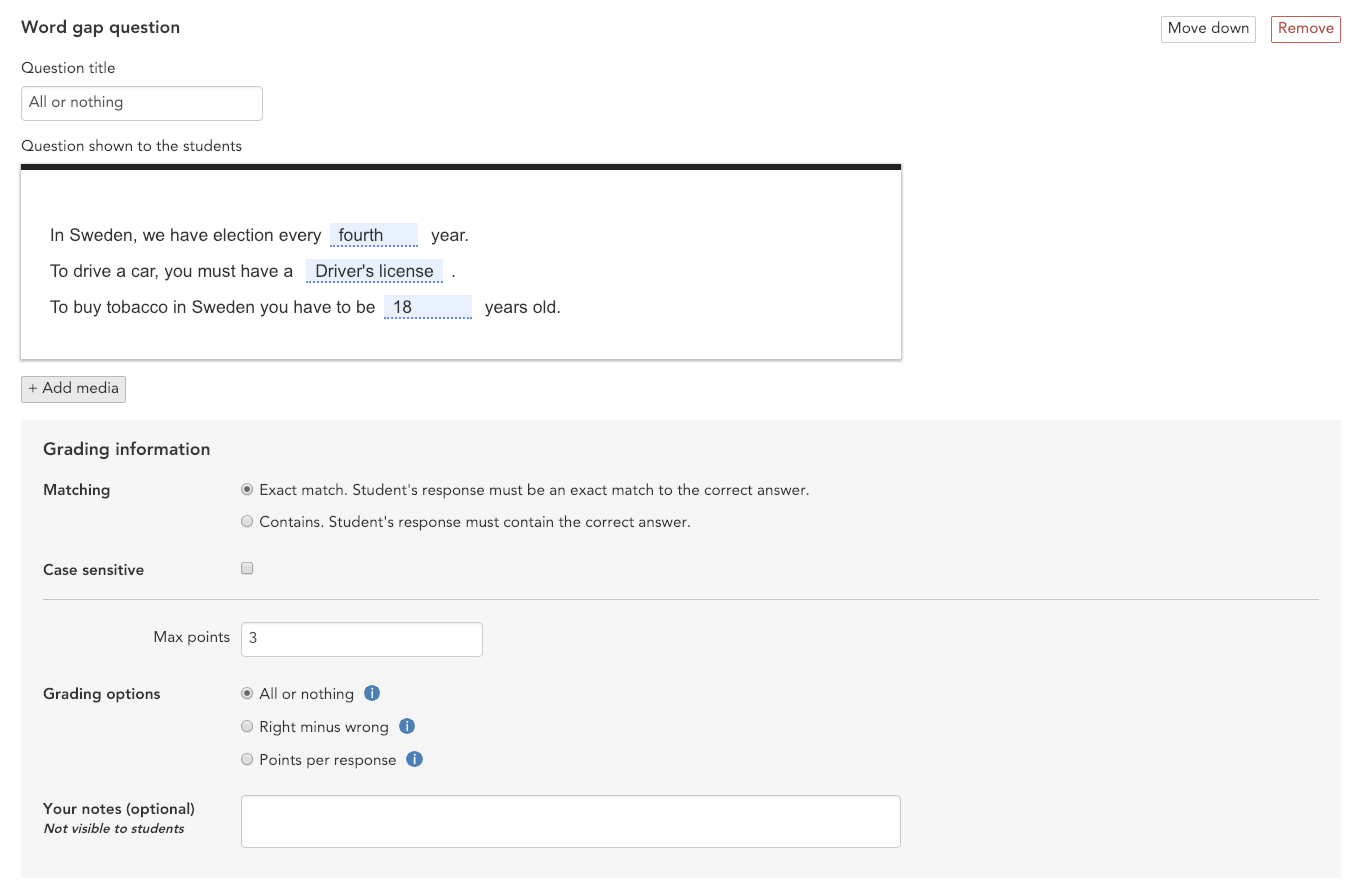Figure 1

The examples below are based on the word gaps in Figure 1.

Example 1: Student 1 writes: fourth, driver's license, 18. Since all answers were correctly written, it will be 3 points.

Example 2: Student 2 writes: 4, driver's license, 18. Since the student did not write "fourth" but instead the number 4, it was wrong and will then be 0 points.

Example 3: Student 3 writes: fourth, driver's license, eighteen. The same goes for this when the student wrote eighteen instead of the numbers 18, so then its 0 points.

Example 4: Student 4 writes: fourth, driver's license-B, 18. Here it was possible to score if the setting "Contains" was chosen instead of "Exact match" which requires it to be exactly as specified in the gap, it will be 0 points.

### Right minus wrong

Students receive plus points for correct spelling and minus points for each incorrect spelling. Each word gap is worth the fraction of the maximum points: So in this case, the maximum score is 4, then every word gap is worth 1 point. So if you write 2 right and 2 wrong then it will be +2 - (-2) = 0 in the final score. The lowest score awarded is zero, so it is not possible to assign minus points (for example -1) as points on "Word gap".

Figure 2 below shows an example of a multiple-choice question with "Contains" and "Case-sensitive" settings.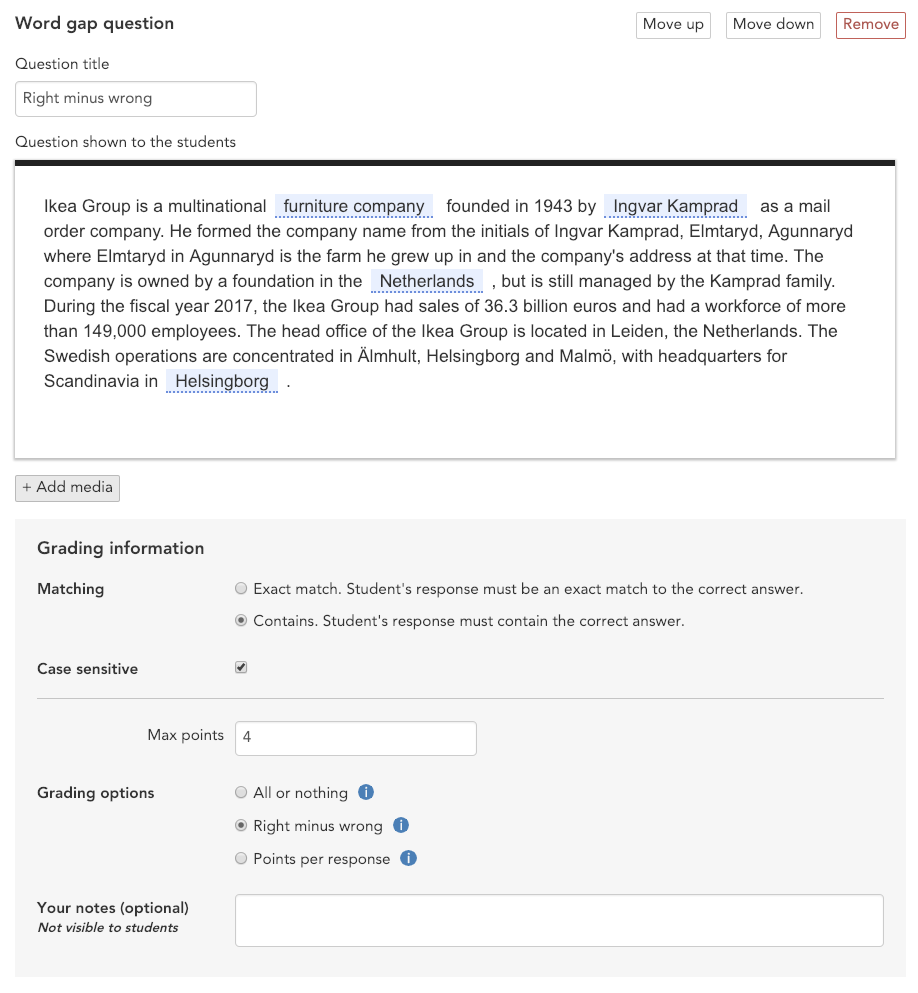Figure 2

The examples below are based on the question in Figure 2.

Example 1: Student 1 writes: furniture company, Ingvar Kamprad, Netherlands, Helsingborg. All words are spelt correctly and had case sensitive letters so it will be 4 points.

Example 2: Student 2 writes: Furniture company, Ingvar kamprad, The Netherlands, Helsingborg. This then becomes 2 points due to 2 wrong case sensitive letters.

Example 3: Student 3 writes: furniture company, ingvar kamprad, netherlands, helsingborg. This will be 0 points when three errors will remove the points that were correct.

Each correct spelling gives plus points and if the spelling of a gap is wrong it won't give any points. Each correct answer is worth the maximum points divided by the number of gaps.

In figure 3 below we have a question worth 5 points with 5 gaps. So 1 point per correct spelling and case sensitive.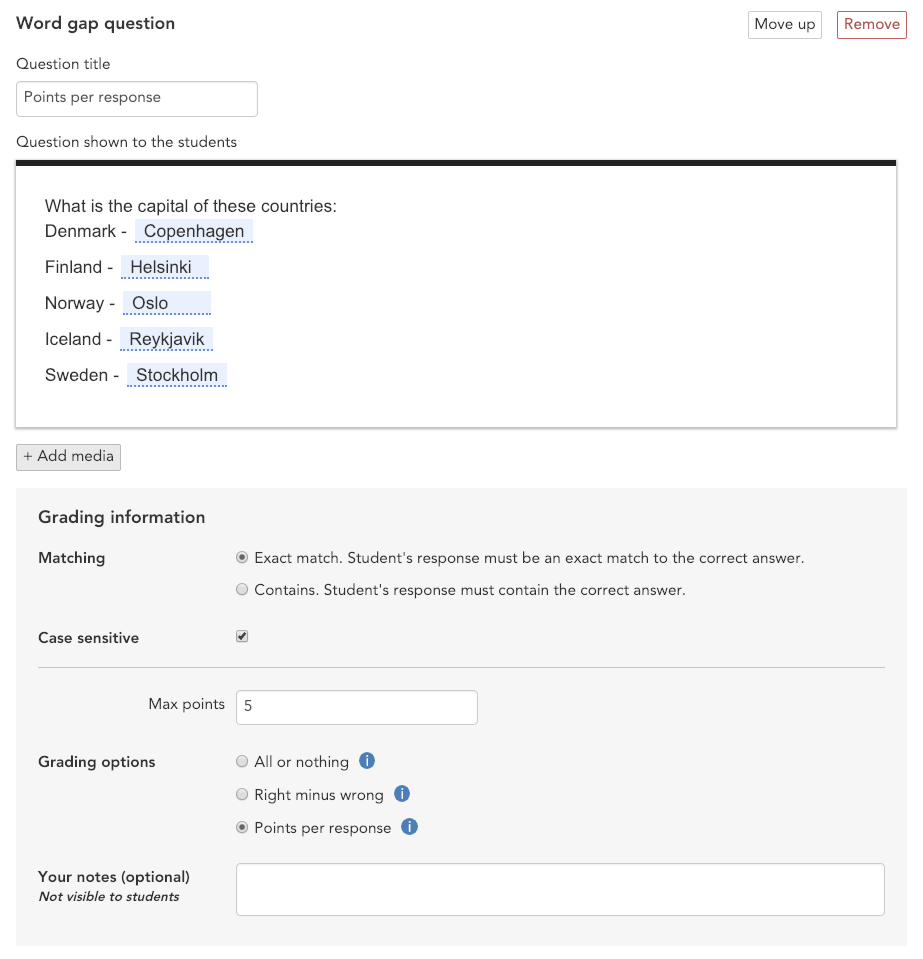Figure 3

## Preview of the exam

With a preview of the exam, you get a picture of what the exam looks like. Numbers will appear indicating the number of the word gap in that view. These are not visible to Pupils / Students.

## Word gap from the Student's perspective

The Student will not see if its "All or nothing", "Right minus error" or "Points per answer". See examples of Word gaps when the student answers them in Figures 3, 4 and 5 below.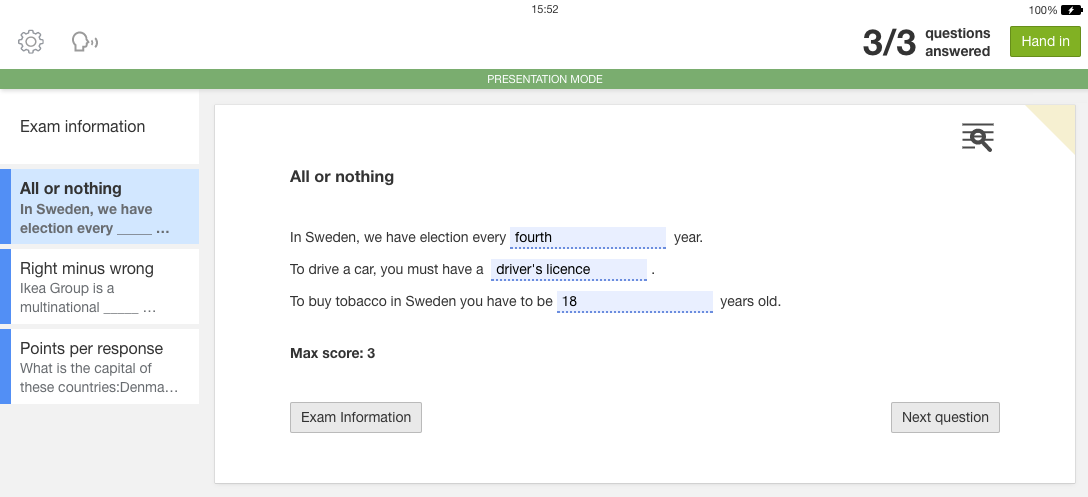Figure 4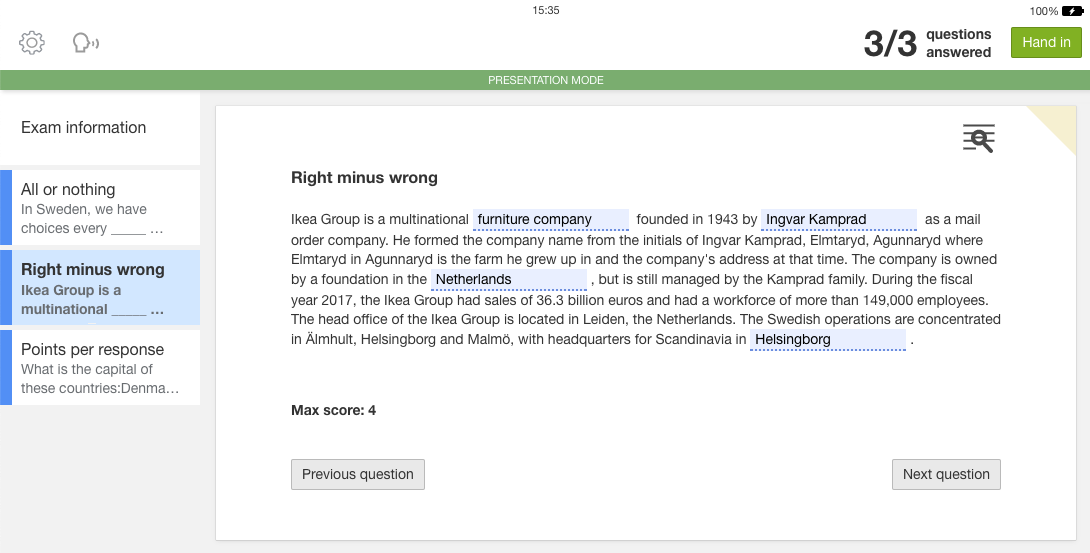Figure 5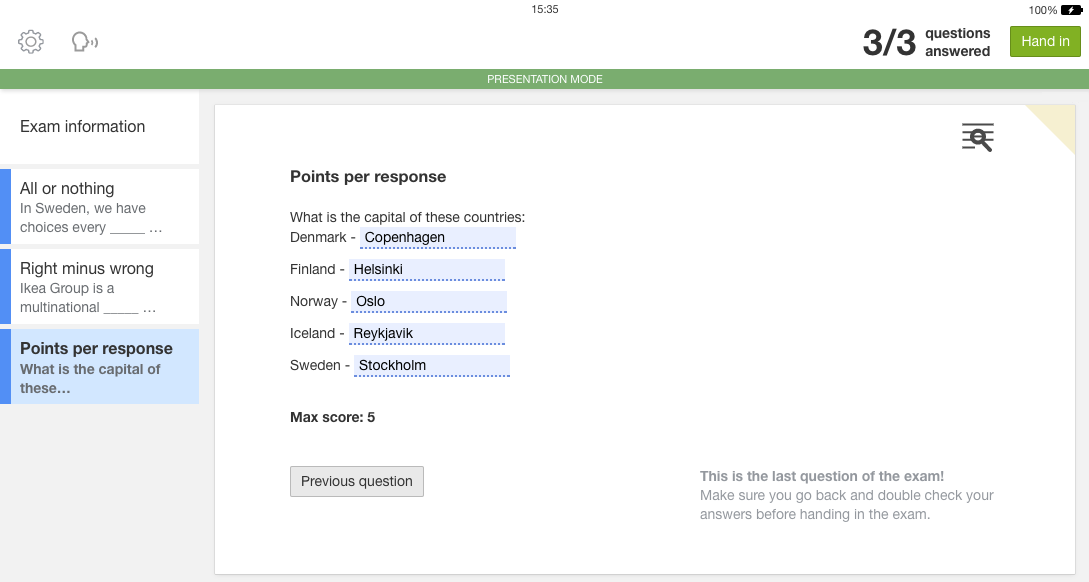Figure 6